# ggplot2: Bar Plots

### Introduction

This is the ninth post in the series Elegant Data Visualization with ggplot2. In the previous post, we learnt to build line charts. In this post, we will learn to:

• build
• simple bar plot
• stacked bar plot
• grouped bar plot
• proportional bar plot
• map aesthetics to variables
• specify values for
• bar color
• bar line color
• bar line type
• bar line size

### Libraries, Code & Data

We will use the following libraries in this post:

All the data sets used in this post can be found here and code can be downloaded from here.

### Data

``````ecom <- read_csv('https://raw.githubusercontent.com/rsquaredacademy/datasets/master/ecom.csv',
col_types = list(col_factor(levels = c('Desktop', 'Mobile', 'Tablet')),
col_factor(levels = c(TRUE, FALSE)), col_factor(levels = c(TRUE, FALSE)),
col_factor(levels = c('Affiliates', 'Direct', 'Display', 'Organic', 'Paid', 'Referral', 'Social'))))
ecom``````
``````## # A tibble: 5,000 x 4
##    device  bouncers purchase referrer
##    <fct>   <fct>    <fct>    <fct>
##  1 Desktop FALSE    FALSE    Affiliates
##  2 Mobile  FALSE    FALSE    Affiliates
##  3 Desktop TRUE     FALSE    Organic
##  4 Desktop FALSE    FALSE    Organic
##  5 Mobile  TRUE     FALSE    Direct
##  6 Desktop TRUE     FALSE    Direct
##  7 Desktop FALSE    FALSE    Referral
##  8 Tablet  TRUE     FALSE    Organic
##  9 Mobile  TRUE     FALSE    Social
## 10 Desktop TRUE     FALSE    Organic
## # ... with 4,990 more rows``````

### Data Dictionary

• device: device used to visit the website
• bouncers: whether visit was a bouncer (exit website from landing page)
• purchase: whether visitor purchased
• referrer: referrer website/search engine

### Aesthetics

• `fill`
• `color`
• `linetype`
• `size`
• `position`

#### Simple Bar Plot

We can create a bar plot using `geom_bar()`. It takes a single input, a categorical variable. In the below example, we plot the number of visits for each device type.

``````ggplot(ecom) +
geom_bar(aes(device))``````#### Bar Color

The color of the bars can be modified using the `fill` argument. In the below example, we assign different colors to the 3 bars in the plot. If you use the `color` argument, it will modify the color of the bar line and not the background color of the bars. We will look at that later in the post.

``````ggplot(ecom) +
geom_bar(aes(device), fill = c('red', 'blue', 'green'))``````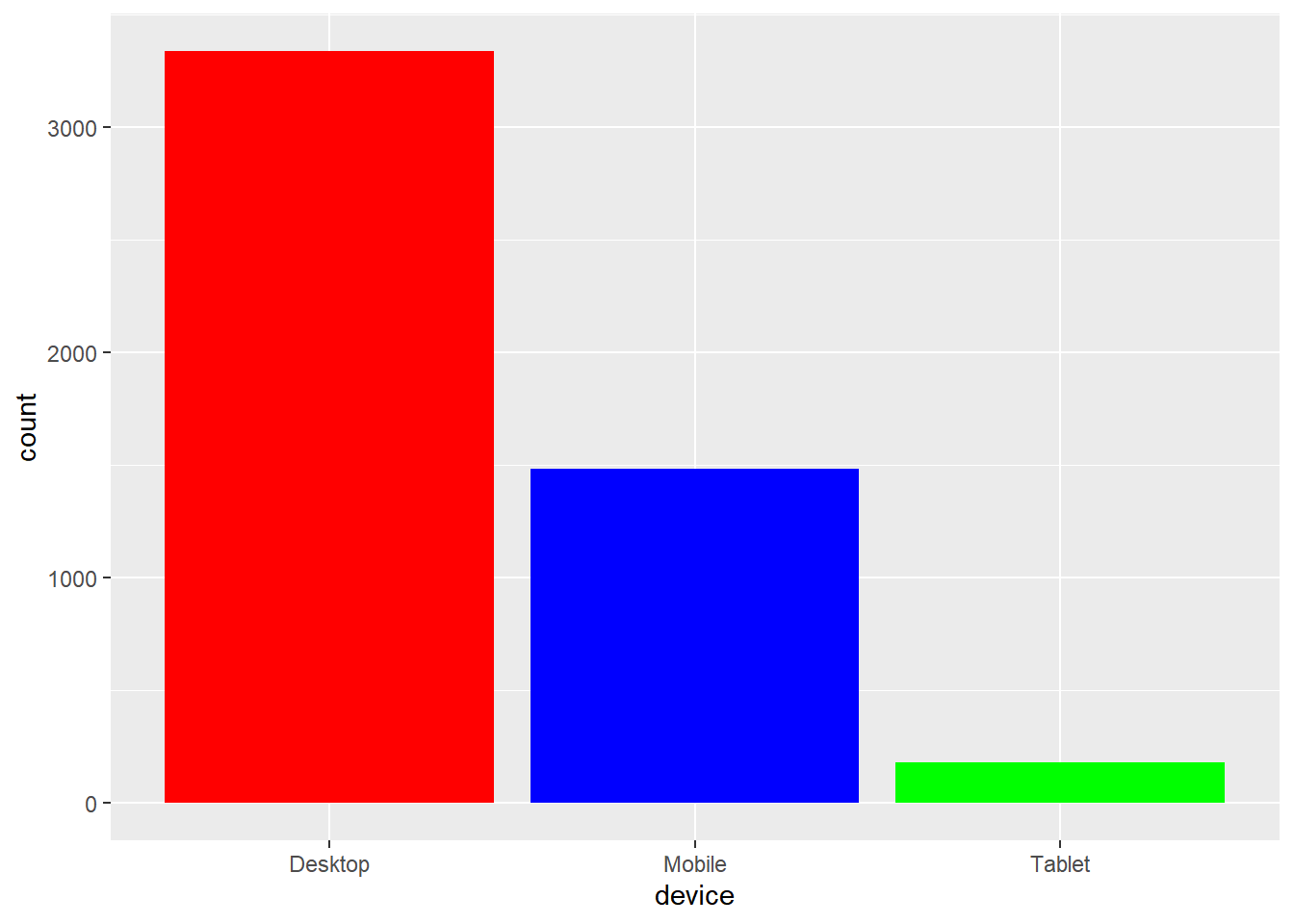#### Stacked Bar Plot

If you want to look at distribution of one categorical variable across the levels of another categorical variable, you can create a stacked bar plot. In ggplot2, a stacked bar plot is created by mapping the `fill` argument to the second categorical variable. In the below example, we have mapped `fill` to `referrer` variable.

``````ggplot(ecom) +
geom_bar(aes(device, fill = referrer))``````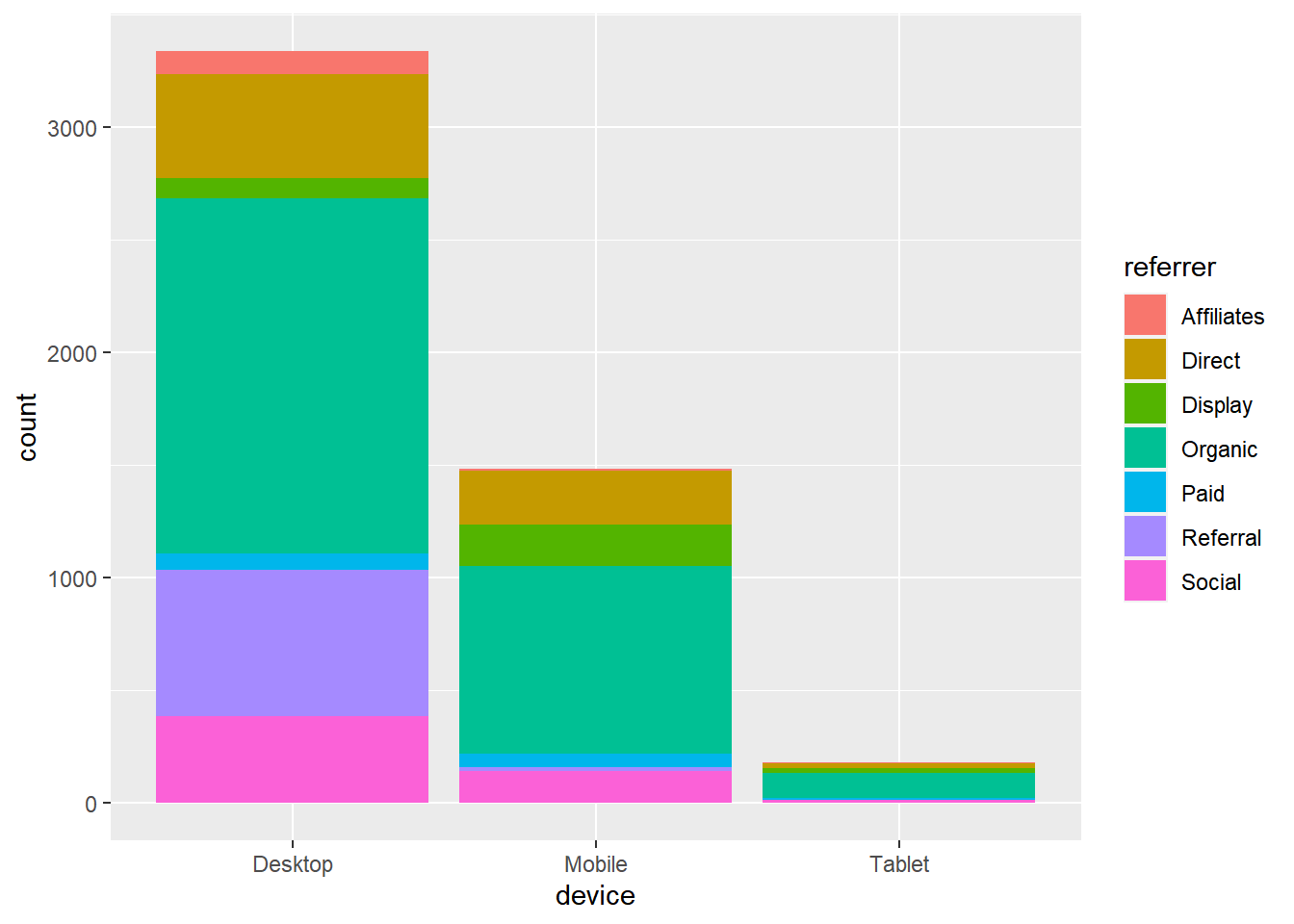#### Grouped Bar Plot

Grouped bar plots are a variation of stacked bar plots. Instead of being stacked on top of one another, the bars are placed next to one another and grouped by levels. In the below example, we create a grouped bar plot and you can observe that the bars are placed next to one another instead of being stacked as was shown in the previous example. To create a grouped bar plot, use the `position` argument and set it to `'dodge'`.

``````ggplot(ecom) +
geom_bar(aes(device, fill = referrer), position = 'dodge')``````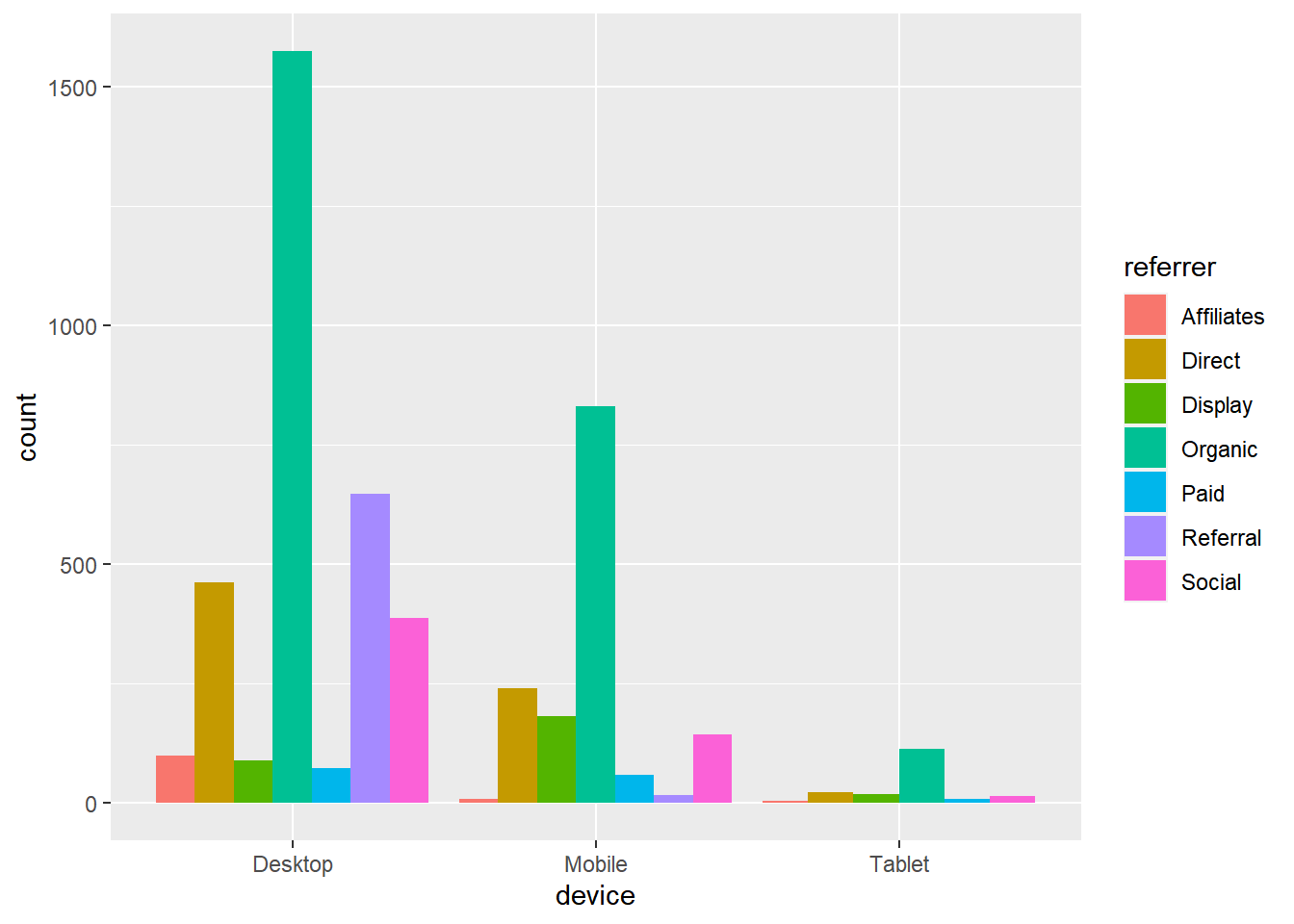#### Proportional Bar Plot

In a proportional bar plot, the height of all the bars is proportional or same. To create a proportional bar plot, use the `position` argument and set it to `'fill'`.

``````ggplot(ecom) +
geom_bar(aes(device, fill = referrer), position = 'fill')``````#### Horizontal Bar Plot

A horizontal bar plot can be created by flipping the coordinate axes of a regular plot. To flip the axes, use `coord_flip()` as shown below.

``````ggplot(ecom) +
geom_bar(aes(device, fill = referrer)) +
coord_flip()``````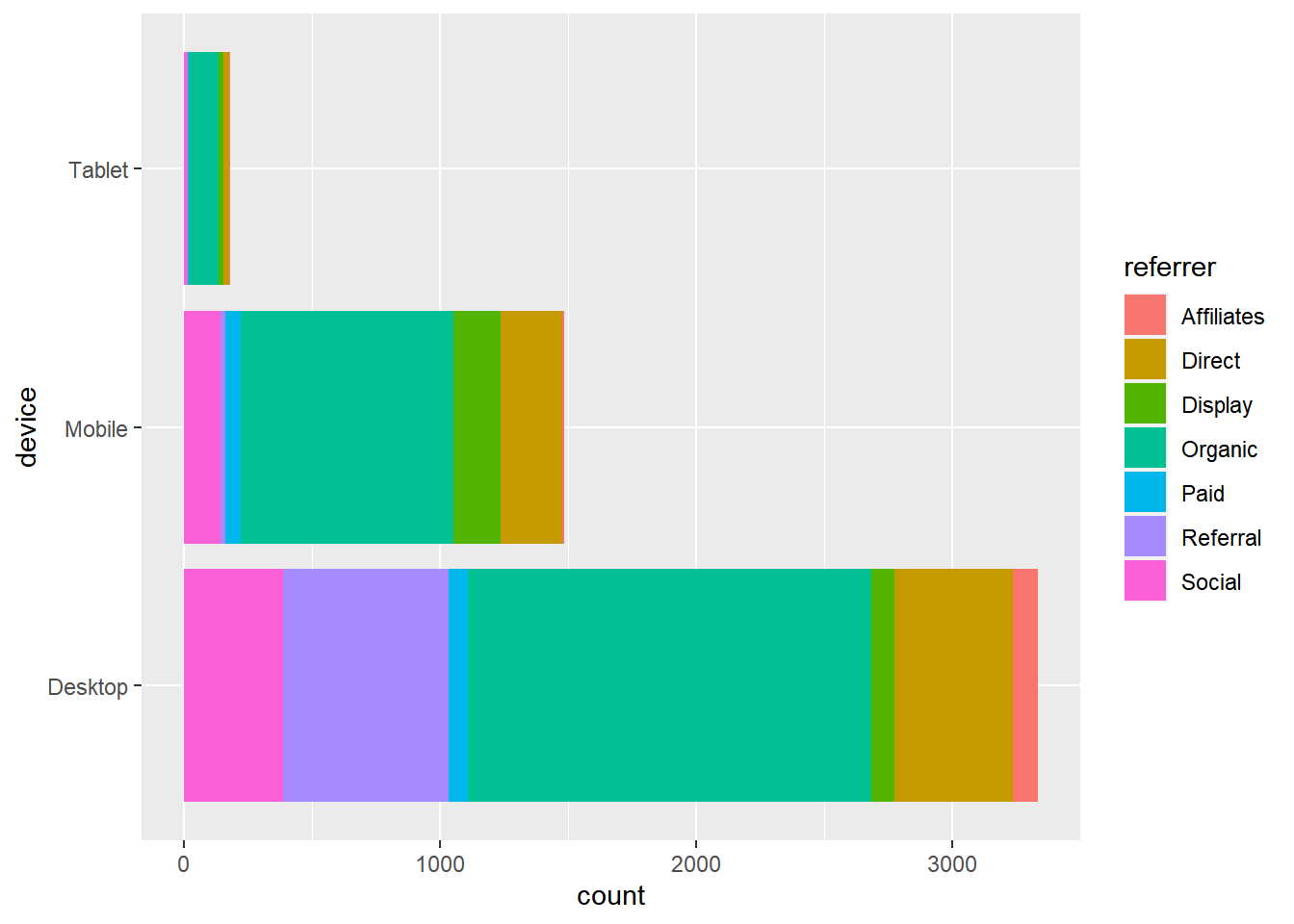#### Bar Line

The color of the bar line can be modified using the `color` argument. The color can be specified either using its name or hex code.

``````ggplot(ecom) +
geom_bar(aes(device), fill = 'white', color = c('red', 'blue', 'green'))``````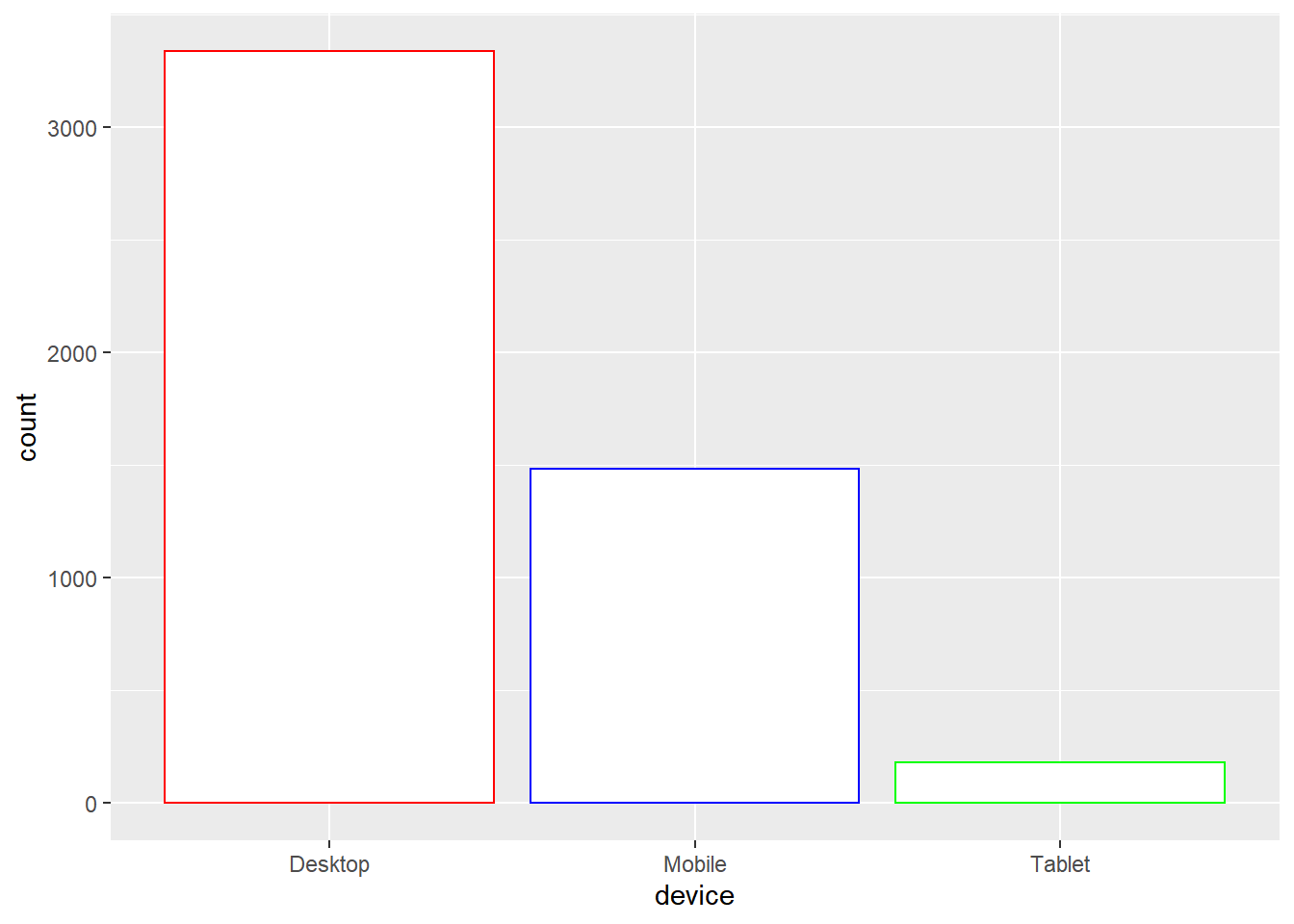To modify the line type of the bar line, use the `linetype` argument. It can take values between `0` and `6`.

``````ggplot(ecom) +
geom_bar(aes(device), fill = 'white',  color = 'black', linetype = 2)``````The width of the bar line can be modified using the `size` argument. It can take any value greater than `0`.

``````ggplot(ecom) +
geom_bar(aes(device), fill = 'white', color = 'black', size = 2)``````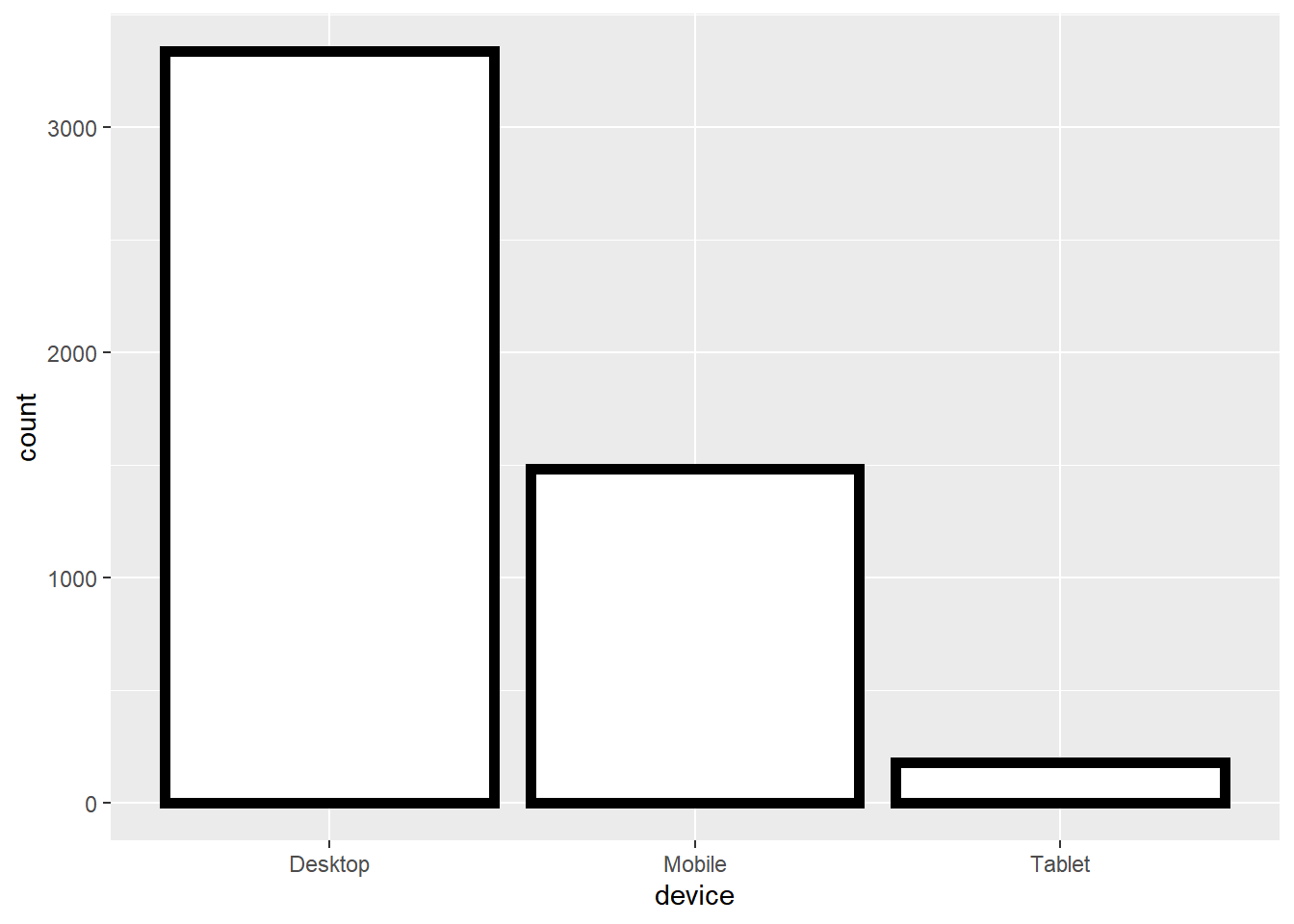### Summary

In this post, we learnt to:

• build
• simple bar plot
• stacked bar plot
• grouped bar plot
• proportional bar plot
• map aesthetics to variables
• specify values for
• bar color
• bar line color
• bar line type
• bar line size

### Up Next..

In the next post, we will learn to build box plots.